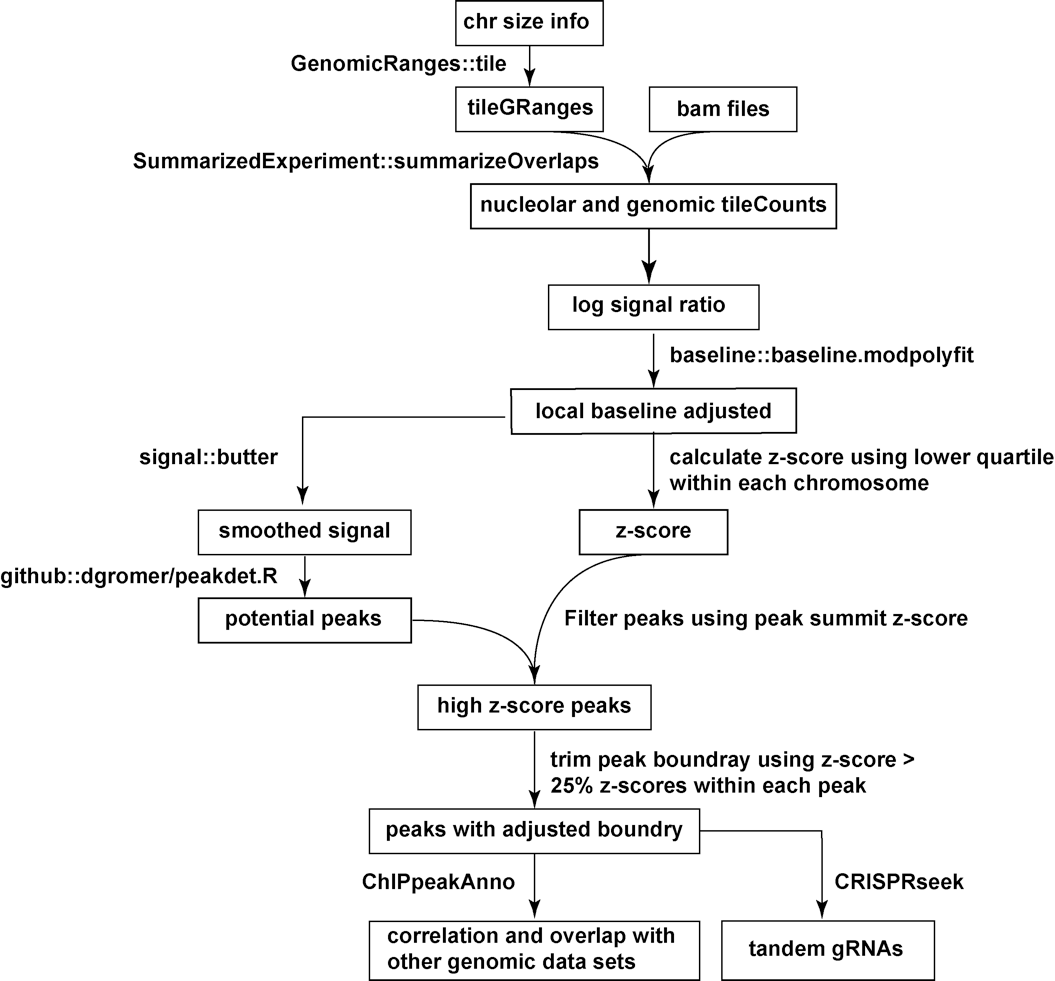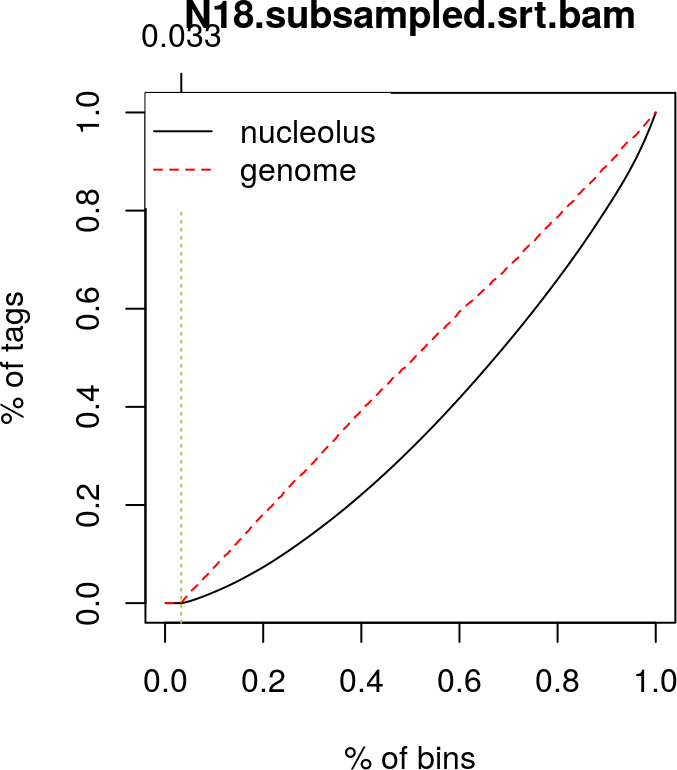# 1 Introduction

Nucleoli serve as major organizing hubs for the three-dimensional structure of mammalian heterochromatin1. Specific loci, termed nucleolar-associated domains (NADs), form frequent three-dimensional associations with nucleoli2. Early mammalian development is a critical period to study NAD biological function, because interactions between pericentromeric chromatin and perinucleolar regions are particularly dynamic during embryonic development4. Here, we developed a Bioconductor package NADfinder for bioinformatic analysis of the NAD-seq data, including normalization, smoothing, peak calling, peak trimming and annotation. We demonstrate the application of NADfinder in mapping the NADs in the mouse genome, determining how these associations are altered during embryonic stem cell (ESC) differentiation, and develop tools for study of these higher-order chromosome interactions in fixed and live single cells (Fig. 1).Fig. 1 Workflow for NAD peak identification. Read counts are summarized for each 50kb moving window with step size of 1kb for nucleolar and genomic samples. Log2 ratio between nucleolar and genomic samples was computed for each window, followed by local background correction, smoothing, peak calling, filtering and annotation.

# 2 Single pair of nucleoleus associated DNA and whole genomic DNA sequencing

samples
Here is an example to use NADfinder for peak calling.

## 2.1 Coverage calculation

Here is the code snippet for calculating coverage with a sliding window in a given step along the genome using a pair of bam files from genomic sample as background and purified nucleoleus-associated DNA as target signal.

``````## load the library
library(SummarizedExperiment)
## test bam files in the package
path <- system.file("extdata", package = "NADfinder", lib.loc = NULL,
mustWork = TRUE)
bamFiles <- dir(path, ".bam\$")

## window size for tile of genome. Here we set it to a big window size,
## ie., 50k because of the following two reasons:
## 1. peaks of NADs are wide;
## 2. data will be smoothed better with bigger window size.
ws <- 50000
## step for tile of genome
step <- 5000
## Set the background.
## 0.25 means 25% of the lower ratios will be used for background training.
backgroundPercentage <- 0.25
## Count the reads for each window with a given step in the genome.
## The output will be a GRanges object.
library(BSgenome.Mmusculus.UCSC.mm10)``````
``````se <- tileCount(reads=file.path(path, bamFiles),
genome=Mmusculus,
windowSize=ws,
step=step,
mode = IntersectionNotStrict,
dataOverSamples = FALSE)``````

Here we load the pre-computed coverage data single.count to save build time.

``````data(single.count)
se <- single.count``````

For quality asessment, `cumulativePercentage` can be used to plot the cumulative sums of sorted read counts for nucleolus-associated DNA and whole genomic DNA. We expect the cumulative sum in the genomic DNA to be close to a straight line because the coverage for the genomic DNA sample should be uniformly distributed. Unlike ChIP-seq data, the cumulative sum for the nucleoleus sample will not exhibit sharp changes because most of NADs are broad regions as wide as 100 kb. However, we should observe clear differences between the two curves.

``````## Calculate ratios for peak calling. We use nucleoleus vs genomic DNA.
dat <- log2se(se, nucleolusCols = "N18.subsampled.srt.bam",
genomeCols ="G18.subsampled.srt.bam",
transformation = "log2CPMRatio")
## Smooth the ratios for each chromosome.
## We found that for each chromosome, the ratios are higher in 5'end than 3'end.
datList <- smoothRatiosByChromosome(dat, chr = c("chr18", "chr19"), N = 100)
## check the difference between the cumulative percentage tag allocation
## in genome and nucleoleus samples.
cumulativePercentage(datList[["chr18"]])``````## 2.2 Call peaks

Before peak calling, the function `smoothRatiosByChromosome` is used for log ratios calculation, normalization and smoothing.

The peaks will be called if the ratios are significantly higher than chromosome-specific background determined by `trimPeaks`. The following figure shows the peaks (black rectangles) called using normalized (green curve) and smoothed (red curve) log2 ratios.

``````## check the results of smooth function
chr18 <- datList[["chr18"]] ## we only have reads in chr18 in test data.
chr18subset <- subset(chr18, seq.int(floor(length(chr18)/10))*10)
chr18 <- assays(chr18subset)
ylim <- range(c(chr18\$ratio[, 1],
chr18\$bcRatio[, 1],
chr18\$smoothedRatio[, 1]))
plot(chr18\$ratio[, 1],
ylim=ylim*c(.9, 1.1),
type="l", main="chr18")
abline(h=0, col="cyan", lty=2)
points(chr18\$bcRatio[, 1], type="l", col="green")
points(chr18\$smoothedRatio[, 1], type="l", col="red")
legend("topright",
legend = c("raw_ratio", "background_corrected", "smoothed"),
fill = c("black", "green", "red"))
## call peaks for each chromosome
peaks <- lapply(datList, trimPeaks,
backgroundPercentage=backgroundPercentage,
cutoffPvalue=0.05, countFilter=1000)
## plot the peaks in "chr18"
peaks.subset <- countOverlaps(rowRanges(chr18subset), peaks\$chr18)>=1
peaks.run <- rle(peaks.subset)
run <- cumsum(peaks.run\$lengths)
run <- data.frame(x0=c(1, run[-length(run)]), x1=run)
#run <- run[peaks.run\$values, , drop=FALSE]
rect(xleft = run\$x0, ybottom = ylim*.75,
xright = run\$x1, ytop = ylim*.8,
col = "black")``````